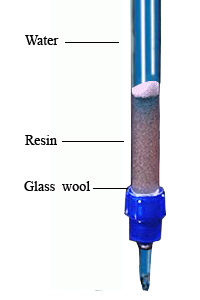# Determination of total amount of cations in water

Different types of metal salt are dissolved in natural, industrial or any other water. Therefore, the water contains different type of cations. The cations in water can be determined by using cation exchange resin. They are electron donors and consequent positively charged. Some common cations are Na+, K+, Mg2+, Ca2+, Fe2+ or Fe3+, Mn2+, Al3+. On the other hand, cation exchange resin contains negatively charged group. The most common group is sulfonate group, -SO3. If the water passes through a cation exchange resin in hydrogen form then the resin except the metal ion from the water and leave equivalent hydrogen ion. In other words leave an equivalent amount of acid. It is just a substitution reaction.

M+ + R-H → R-M + H+

This total amount of acid can be determined by titration with NaOH solution.Regeneration
Initially the cation exchanger resin may be regenerated to introduce hydrogen ion into the resin. To regenerate a column, you have to pass HCl through the cation exchange resin. Then the following reaction occurs which is revise process of previous reaction.
R-M + HCl → R-H + MCl
Additional acid and metal salt are removed from resin by passing cation free water (distilled water).

Apparatus Required:

• Cation exchange resin column
• 250ml volumetric flasks
• 20ml, 25ml pipette
• Beakers
• Graduated cylinder
• pH meter and pH electrode
• Wash bottle

Chemicals:

• 2N HCl
• Methyl orange indicator
• AgNO3 solution
• distilled water
• litmus paper

Procedure:

1. Took a long glass tube (as like burette) with stopcock and make a 25-30 ml long column by keeping some glass wool at the end point of the glass tube.
2. Pass 250 ml 2N HCl through the column so that 3-4 ml solution is passing per minutes.
3. Then rinse the column with distilled water until the elution becomes neutral (no acidity or pH is greater than 5.8). You can cheek it by the methyl orange or pH meter. Moreover, you can also use AgNO3 solution to check for the presence of Cl ion; it indicates the presence of HCl. It should be noticed that always some water exist on the column.
4. Take about 40 ml sample water with the pipette and pass 3-4 ml water per minutes through the column. Exit the elution and mark the upper level of the resin column.
5. Now take 20ml sample water, then pass it through the column at same rate and collect the elution in a conical flask. At the moment, it should be noticed that the upper level of the resin column remain equal.
6. Collect two more at same process.
7. This resulting H+ (the collected water) can be determined by titration with NaOH using methyl orange indicator. Then calculated the original concentration of cations in water using the formula.

You can direct use the sample water. It may require diluted the water in case of higher concentration of cations. To dilute take 25ml sample water in a 250ml volumetric flask and fill up to the mark with distilled water.

Calculation
Generally, the total amount of cation in water is expressed as equivalent mineral acidity (EMA). It is the amount of equivalent calcium carbonate of per liter solution. If the volume of sample water is V ml, normality of the NaOH is B and the volume of the NaOH is A ml, then
EMA = (AᵡBᵡ50ᵡ1000)/V

## 1 thought on “Determination of total amount of cations in water”

1.prafullo kumar maharana says:

EMA = (AᵡBᵡ50ᵡ1000)/V
clearly specify use and introduce of digit ’50’ and ‘1000’ in formula from where they applied in experiment period and amount of total cations express in gram or milligram unit if.